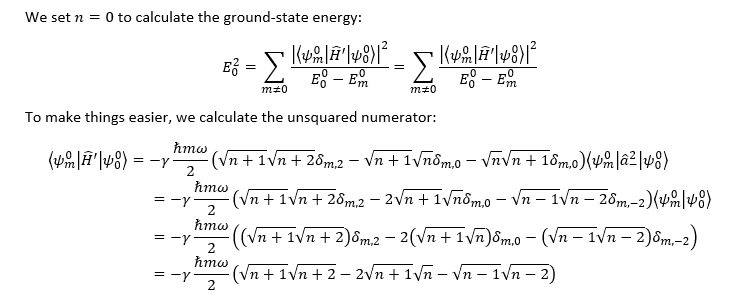# ND Pertubation theory: Second order correction

• Schwarzschild90

## Homework Statement

Calculate the second-order correction to the ground-state energy of the stationary states of the system.

The perturbed Hamiltonian is:

H' =- (/gamma /hbar m /omega)/2 (a+ - a-) ^2

2 & 3. Relevant equatio and the attempt at a solutionThis is not right. I follow the same procedure as letting the ladder operators act on eigenvalues, i.e. that a+|n> becomes (√(n+1))|n+1>

Last edited:

Did you have a question?

I didn't notice at first that my post is lacking some details.

My question is this: Where in my calculations did I make a mistake in calculating a solution to the problem?

## Homework Statement

Calculate the second-order correction to the ground-state energy of the stationary states of the system.

The perturbed Hamiltonian is:

H' =- (/gamma /hbar m /omega)/2 (a+ - a-) ^2

2 & 3. Relevant equatio and the attempt at a solution
View attachment 101566
This is not right. I follow the same procedure as letting the ladder operators act on eigenvalues, i.e. that a+|n> becomes (√(n+1))|n+1>
Why do you have factors of "n" all over the place? There should be only an "m" and in each term is has a specific value (like m=2!) so you should get numbers for all the matrix elements (and most of them are zero)

How do I figure out which value of m each matrix element should have?

I figured it out.

I got the following result: sqrt(1)sqrt(2).

(Which is correct)